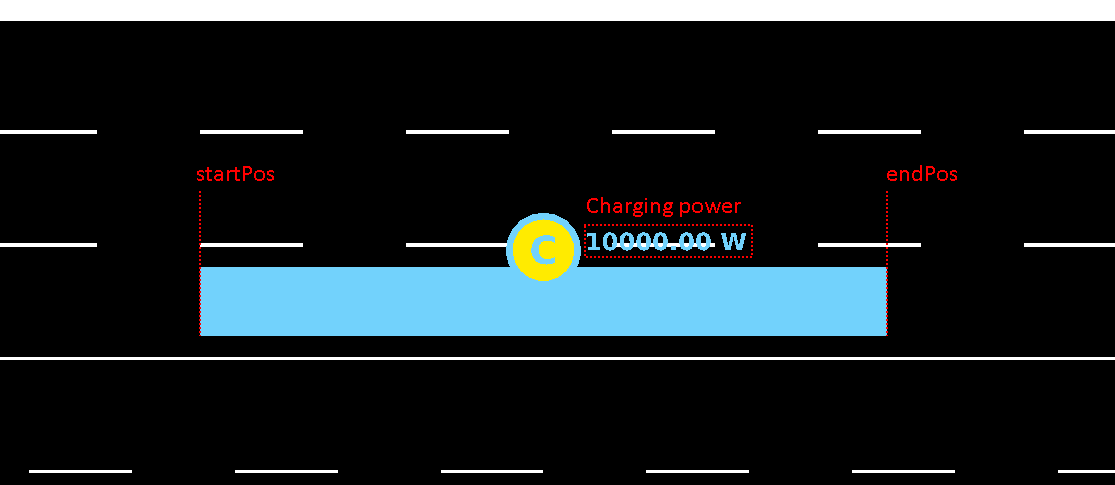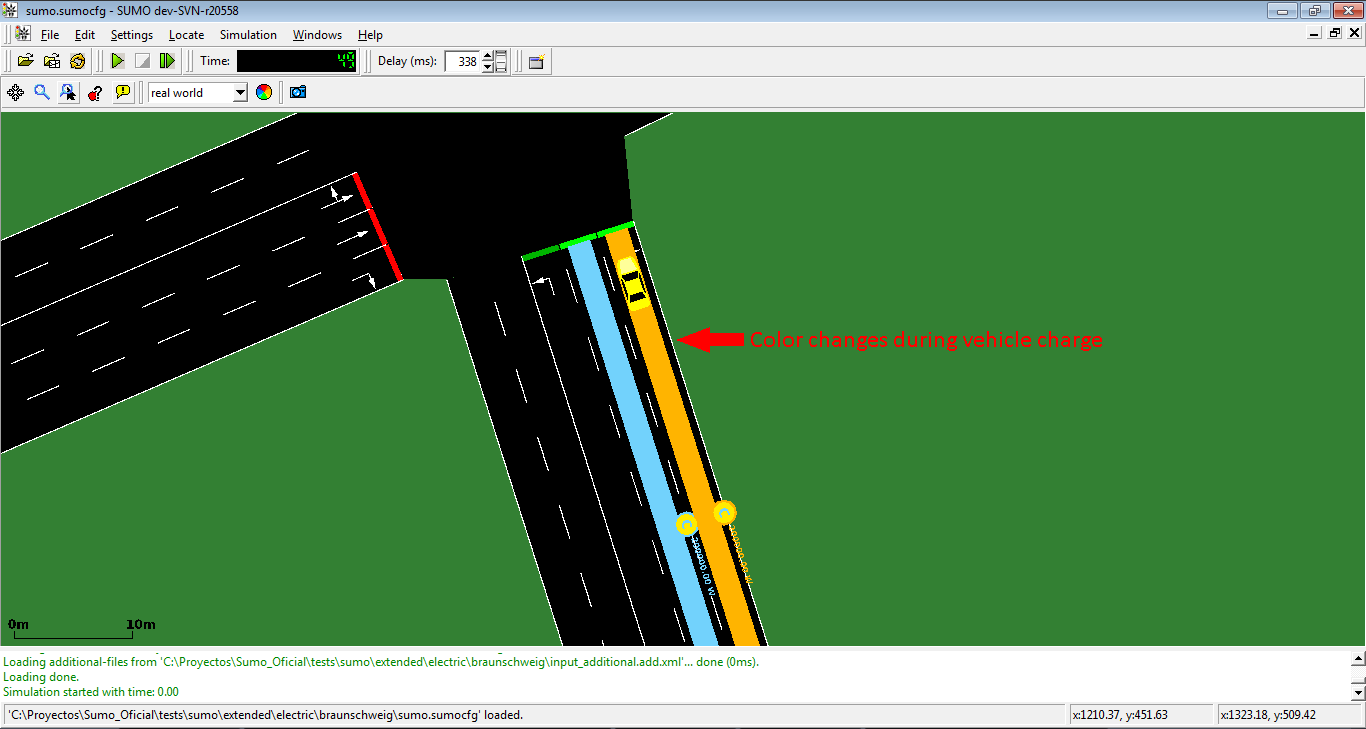Models/Electric

# Overview#

Since version 0.24.0 SUMO includes a model for electric vehicles. It was implemented by Tamás Kurczveil and Pablo Álvarez López from the TU-Braunschweig. The core of the model is implemented in the vehicle device device.battery. Additional features are a charging station (which can be placed on any lane in the network) and a new output option --battery-output <FILE>.

You can find a test case for these implementations at 

## Defining Electric Vehicles#

To define an electric vehicle, it must be equipped with a battery device. This may be done using the option --device.battery.explicit <vehID1,vehID2,...> or simply setting --device.battery.probability 1 to equip all vehicles. Alternatively, the device may be specified using Generic vehicle parameters.

Additional properties of the vehicle and its electrical components must then be defined via parameters of the vehicle or its type.

These values have the following meanings:

key Value Type Default Description
maximumBatteryCapacity float 0 (Wh) Maximum battery capacity Emax
maximumPower float 0 (W) Maximum power which the vehicle can reach
vehicleMass float 0 (kg) Vehicle mass mveh
frontSurfaceArea float 0 (m2) Front surface area Aveh
airDragCoefficient float 0 Air drag coefficient cw
internalMomentOfInertia float 0 (kg·m2) Mom. of inertia of int. rot. elements Jint
rollDragCoefficient float 0 Rolling resistance coefficient croll
constantPowerIntake float 0 (W) Avg. (constant) power of consumers Pconst
propulsionEfficiency float 0 Drive efficiency ηprop
recuperationEfficiency float 0 Recuperation efficiency ηrecup
stoppingTreshold float 0.1 (km/h) Minimum velocity to start charging

An example of a vehicle with electric attribute:

``````<routes>
<vType id="ElectricBus" accel="1.0" decel="1.0" lenght="12" maxSpeed="100.0" sigma="0.0" minGap="2.5" color="1,1,1">
<param key="has.battery.device" value="true"/>
<param key="maximumBatteryCapacity" value="2000"/>
<param key="maximumPower" value="1000"/>
<param key="vehicleMass" value="10000"/>
<param key="frontSurfaceArea" value="5"/>
<param key="airDragCoefficient" value="0.6"/>
<param key="internalMomentOfInertia" value="0.01"/>
<param key="rollDragCoefficient" value="0.01"/>
<param key="constantPowerIntake" value="100"/>
<param key="propulsionEfficiency" value="0.9"/>
<param key="recuperationEfficiency" value="0.9"/>
<param key="stoppingTreshold" value="0.1"/>
</vType>
</routes>
``````

The initial energy content of the battery (by default MaxBatKap/2) can be set in the vehicle definitions

``````<routes>
<vehicle id="0" type="type1" depart="0" color="1,0,0">
<param key="actualBatteryCapacity" value="500"/>
</vehicle>
</routes>
``````

## Charging Stations#

A charging station is a surface defined on a lane in which the vehicles equipped with a battery are charged. The basic structure and parameters of bus stops were used for the implementation of charging stations.

key Value Type Value range Default Description
id string id Charging station ID (Must be unique)
lane string valid lane id Lane of the charging station location
startPos float lane.length < x < lane.length (negative values count backwards from the end of the lane) 0 Begin position in the specified lane
endPos float lane.length < x < lane.length (negative values count backwards from the end of the lane) End position in the specified lane
power float power > 0 22000(Wh) Charging power Pchrg
efficiency float 0 <= efficiency <= 1 0.95 Charging efficiency ηchrg
chargeInTransit bool 0 or 1 0 Enable or disable charge in transit, i.e. vehicle is forced/not forced to stop for charging
chargeDelay float chargeDelay > 0 0 Time delay after the vehicles have reached / stopped on the charging station, before the energy transfer (charging) is starting
name string simple String Charging station name. This is only used for visualization purposes.

Charging stations are defined in additional using the following format:

``````<additional>
<chargingStation chargeDelay="2" chargeInTransit="0" chrgpower="200000" efficiency="0.95" endPos="25" id="cS_2to19_0a" lane="2to19_0" startPos="10"/>
``````

And are represented in the simulation as shown:Representation of chargingStation in GUIColor of chargingStation during charge

### Stopping at a Charging Station#

A stop at a charging station may occur due to traffic conditions, stopping at a defined location or stopping at an explicit chargingStation as defined below:

``````<routes>
<vehicle id="v0" route="route0" depart="0">
<stop chargingStation="myChargingStationID" until="50"/>
</vehicle>
</routes>
``````

### Charging Station output#

Option --chargingstations-output "nameOfFile.xml" generates a full report of energy charged by charging stations:

``````<output>
<battery-output value="battery.xml"/>
<chargingstations-output value="chargingstations.xml"/>
</output>
``````

File chargingstations.xml has the following structure:

``````<chargingstations-export>
<chargingStation id="CS1" totalEnergyCharged="71.25" chargingSteps="27">
<vehicle id="veh0" type="ElectricVehicle1" totalEnergyChargedIntoVehicle="15.83" chargingBegin="12.00" chargingEnd="17.00">
<step time="12.00" chargingStatus="chargingStopped" energyCharged="2.64" partialCharge="2.64" power="10000.00" efficiency="0.95" actualBatteryCapacity="12962.97" maximumBatteryCapacity="35000.00"/>
<step time="13.00" chargingStatus="chargingStopped" energyCharged="2.64" partialCharge="5.28" power="10000.00" efficiency="0.95" actualBatteryCapacity="12965.59" maximumBatteryCapacity="35000.00"/>
<step time="14.00" chargingStatus="chargingStopped" energyCharged="2.64" partialCharge="7.92" power="10000.00" efficiency="0.95" actualBatteryCapacity="12968.22" maximumBatteryCapacity="35000.00"/>
</vehicle>
<vehicle id="veh1" type="ElectricVehicle2" totalEnergyChargedIntoVehicle="5.28" chargingBegin="17000" chargingEnd="18000">
<step time="17.00" chargingStatus="chargingStopped" energyCharged="2.64" partialCharge="18.47" power="10000.00" efficiency="0.95" actualBatteryCapacity="11967.35" maximumBatteryCapacity="35000.00"/>
<step time="18.00" chargingStatus="chargingStopped" energyCharged="2.64" partialCharge="21.11" power="10000.00" efficiency="0.95" actualBatteryCapacity="12978.72" maximumBatteryCapacity="35000.00"/>
</vehicle>
...
</chargingStation>
...

...
</chargingstations-export>
``````

For the entire ChargingStation:

Name Type Description
id string ChargingStation ID
totalEnergyCharged float Total energy charged in W during the entire simulation
chargingSteps int Number of steps in which charginStation was charging energy

For the current charging vehicle

Name Type Description
id string ID of vehicle that is charging in these charging stop
type string type of vehicle
totalEnergyChargedIntoVehicle double Energy charged during these charging stop
chargingBegin float TimeStep in which vehicle starts with the charge (in seconds)
chargingEnd float TimeStep in which vehicle ends with the charge (in seconds)

For every charging timeStep:

Name Type Description
time float Current timestep (s)
chargingStatus string Current charging status (Charging, waiting to charge o not charging)
energyCharged float Energy charged in current timeStep
partialCharge float Energy charged by ChargingStation from begin of simulation to this timeStep
power float Current power of ChargingStation
efficiency float Current efficiency of ChargingStation
actualBatteryCapacity string Current battery capacity of vehicle
maximumBatteryCapacity string Current maximum battery capacity of vehicle

## battery-output#

There are three output parameters to be set in the SUMO configuration to use the battery device:

``````<configuration>
<input>
<net-file value="netFile.xml"/>
<route-files value="routeFile.xml"/>
</input>
<output>
<battery-output value="Battery.out.xml"/>
<battery-output.precision value="4"/>
<device.battery.probability value="1"/>
<summary-output value="summary_100.xml"/>
</output>
</configuration>
``````

The battery-output generates a file with this structure:

``````<battery-export>
<timestep time="0.00">
<vehicle id="vehicle01" Consum="0.00" actualBatteryCapacity="17500.00" maximumBatteryCapacity="35000.00"
chargingStationId="NULL" energyCharge="0.00" energyChargedInTransit="0.00" energyChargedStopped="0.00"
speed="12.92" acceleration="0.00" x="1428.27" y="25.57" lane="01to02_0"
posOnLane="0.00" timeStopped="0"/>
<vehicle id=..... */
</timestep>
<timestep time="1.00">
<vehicle id=.....
</timestep>
<timestep time=...
...
</timestep>
</battery-export>
``````
Name Type Description
time int Current timestep
id string id of vehicle
energyConsumed double energy consumption in this timestep in Wh
actualBatteryCapacity double energy content of the battery in this timestep
maximumBatteryCapacity double Max energy capacity of the battery
chargingStationId string If vehicle is exactly at a charging station, this value is the id of the charging station, in other case, is NULL
energyCharged double Charge received in the current time step from a charging station (Only != 0 if vehicle is exactly at a charging station)
energyChargedInTransit double Charge that a vehicle in transit received in the current time step from a charging station
energyChargedStopped double Charge that a stopped vehicle received in the current time step from a charging station
speed double Speed of vehicle in this timestep
acceleration double Acceleration of vehicle in this timestep
x double absolute position x of vehicle in the map
y double absolute position y of vehicle in the map
lane string id of the lane that the vehicle is currently on
posOnLane double Position of vehicle on its current lane
timeStopped int Counter with the number of timesteps that the vehicle has remained standing

## Emission Output#

The Emission model-outputs of SUMO can be used together with the battery device when setting the `<vType>`-parameter `emissionClass="Energy/unknown"`.

## TraCI#

The internal state of the battery device can be accessed directly using traci.vehicle.getParameter and traci.vehicle.setParameter.

Furthermore, the function traci.vehicle.getElectricityConsumption() can be used to access the consumption of the vehicle if the `emissionClass="Energy/unknown"` is declared.

## Model Details#

All information about the implemented device (including details on the vehicle energy consumption and charging model) can be found in the following publication.